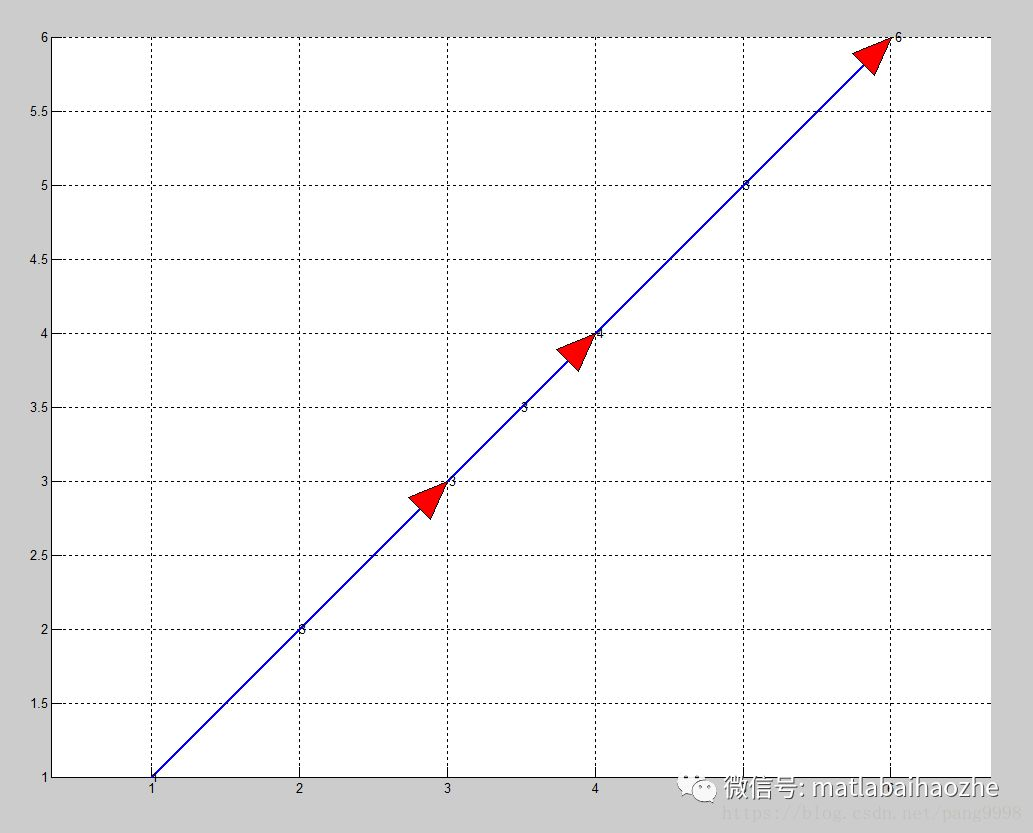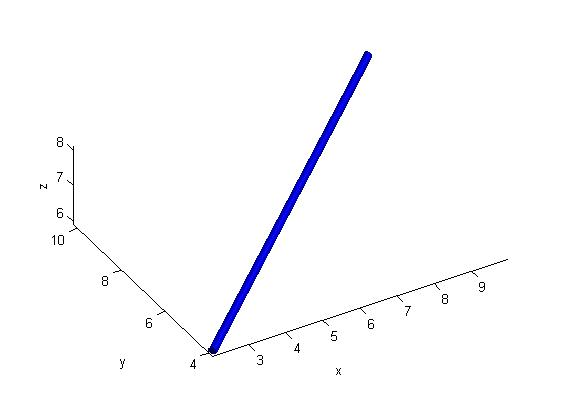• matlab开发-两点之间的距离。通过卡尔森模型计算两个GPS坐标之间的距离。
• Matlab任意两点之间绘制带箭头的直线 简单绘制两点任意两点之间。本来不像自己写的，可是网上的代码用起来不舒服，所以简单看看原理，原来就是个坐标变换而已。索性自己写了一份，分享如下： function drawarrow(x,y...
Matlab任意两点之间绘制带箭头的直线
简单绘制任意两点之间。本来不想自己写的，可是网上的代码用起来不舒服，所以简单看看原理，原来就是个坐标变换而已。索性自己写了一份，分享如下：
function drawarrow(x,y,lineType,ax)
switch nargin
case 2
lineType='arrow';
ax=gca;
case 3
ax=gca;
end
% 调整坐标大小以适应箭头长度
xlim=ax.XLim;
ylim=ax.YLim;
xlimmin=xlim(1);xlimmax=xlim(2);
ylimmin=ylim(1);ylimmax=ylim(2);
if xlimmin>min(x(1),y(1)), xlimmin=min(x(1),y(1));end
if xlimmax<max(x(1),y(1)), xlimmax=max(x(1),y(1));end
if ylimmin>min(x(2),y(2)), ylimmin=min(x(2),y(2));end
if ylimmax<max(x(2),y(2)), ylimmax=max(x(2),y(2));end
ax.XLim = [xlimmin,xlimmax];
ax.YLim = [ylimmin,ylimmax];
xlim=ax.XLim;
ylim=ax.YLim;
pos=ax.Position;
x_ratio = pos(3)/(xlim(2)-xlim(1));
y_ratio = pos(4)/(ylim(2)-ylim(1)); % 缩放比例
orig_pos=[-xlim(1)*x_ratio+pos(1),-ylim(1)*y_ratio+pos(2)]; % figure坐标系中的原点坐标
x=x.*[x_ratio,y_ratio];y=y.*[x_ratio,y_ratio];
x=x+orig_pos;y=y+orig_pos;
annotation(lineType,[x(1),y(1)],[x(2),y(2)])
end

结果如下图中箭头所示：展开全文• matlab开发-计算两点之间的出口循环距离。在给定lat和long的情况下，计算两点之间的大圆距离。
• 若需要精准的坐标，matlab有自带的函数：annotation调用annotation函数绘制二维箭头annotation函数用来在当前图形窗口建立注释对象(annotation对象)，它的调用格式如下：(1) annotation(annotation_...


画箭头，不需要精准位置的话，可以在Figure上的菜单里直接拖拉即可，对应的箭头属性也都可以改。         若需要精准的坐标，matlab有自带的函数：annotation调用annotation函数绘制二维箭头annotation函数用来在当前图形窗口建立注释对象(annotation对象)，它的调用格式如下：(1) annotation(annotation_type)  % 以指定的对象类型，使用默认属性值建立注释对象。(2) annotation('line',x,y)% 建立从(x(1), y(1))到(x(2), y(2))的线注释对象。(3) annotation('arrow',x,y) % 建立从(x(1), y(1))到(x(2), y(2))的箭头注释对象。(4) annotation('doublearrow',x,y)% 建立从(x(1), y(1))到(x(2), y(2))的双箭头注释对象。(5) annotation('textarrow',x,y)  % 建立从(x(1),y(1))到(x(2),y(2))的带文本框的箭头注释对象(6) annotation('textbox',[x y w h])  % 建立文本框注释对象，左下角坐标(x,y)，宽w，高h.(7) annotation('ellipse',[x y w h])  % 建立椭圆形注释对象。(8) annotation('rectangle',[x y w h])% 建立矩形注释对象。(9) annotation(figure_handle,…)     % 在句柄值为figure_handle的图形窗口建立注释对象。(10) annotation(…,'PropertyName',PropertyValue,…)  % 建立并设置注释对象的属性。(11) anno_obj_handle = annotation(…)  % 返回注释对象的句柄值。发现annotation绘制带箭头的直线还挺好用，但是唯一的不足就是需要坐标系在[0,1]范围内的标准坐标系，其他坐标系中绘制会报错!!!       网友发现问题后，自己写的一个可以实现任意俩点绘制箭头的函数，同时颜色和大小都可以修改：%% 绘制带箭头的直线function drawArrow(start_point, end_point,arrColor,lineColor,arrowSize,lineWidth)% 从start_point到end_point画一箭头,arrColor箭头颜色,arrSize，箭头大小%判断参数多少switch nargin    case 2        arrColor  = 'r';        lineColor = 'b';        arrowSize = 2;        lineWidth = 1;    case 3        lineColor = 'b';        arrowSize = 2;        lineWidth = 1;    case 4        arrowSize = 2;        lineWidth = 1;    case 5        lineWidth = 1;endK= 0.05; 箭头比例系数theta= pi / 8;% 箭头角度A1 = [cos(theta), -sin(theta);sin(theta), cos(theta)]; % 旋转矩阵theta = -theta;A2 = [cos(theta), -sin(theta);sin(theta), cos(theta)];% 旋转矩阵arrow= start_point' - end_point';%使得箭头跟直线长短无关(固定值)arrow(arrow>=0)=arrowSize;arrow(arrow<0)=-arrowSize;arrow_1= A1 * arrow;arrow_2= A2 * arrow;arrow_1= K * arrow_1 + end_point'; % 箭头的边的x坐标arrow_2= K * arrow_2 + end_point'; % 箭头的变的y坐标hold on;grid on;axis equal;plot([start_point(1), end_point(1)], [start_point(2), end_point(2)],lineColor,'lineWidth',lineWidth);% 三角箭头(填充)triangle_x= [arrow_1(1),end_point(1),arrow_2(1),arrow_1(1)];triangle_y= [arrow_1(2),end_point(2),arrow_2(2),arrow_1(2)];fill(triangle_x,triangle_y,arrColor);% 线段箭头(不填充)% plot([arrow_1(1), end_point(1)], [arrow_1(2), end_point(2)],color,'lineWidth',arrowSize);% plot([arrow_2(1), end_point(1)], [arrow_2(2), end_point(2)], color,'lineWidth',arrowSize);hold off;end效果如下(亲测实用)：-----本文据网友内容转载而来，供大家学习，原版权如下：作者：pang9998https://blog.csdn.net/pang9998/article/details/82697095电梯直达MATLAB快速入门----处理图形对象Matlab图片水印技术用matlab打造属于自己的密码生成器大话matlabの通用对话框展开全文• Floyd算法求任意两点之间的路径（matlab程序）
• 本函数旨在实现图像中求两点之间的距离 function [dist,phaseangle] = distance(lat,lon,units) % SW_DIST Distance between two lat,lon coordinates %=========================================================...
• 此函数可能存在一些不足，请多多指教！ function plotcylinder(u1,u2,color_a,r) L=norm(u1-u2); ROD=u2-u1; [X,Y,Z]=cylinder(r,100); x1=X*0;y1=Y*0;z1=Z*0; Z=L*Z-L/2; ROD_midpoint=(u1+u2)/2...y=ROD_midpoint(...
此函数可能存在一些不足，请多多指教！
function plotcylinder(u1,u2,color_a,r)
L=norm(u1-u2);
ROD=u2-u1;
[X,Y,Z]=cylinder(r,100);
x1=X*0;y1=Y*0;z1=Z*0;
Z=L*Z-L/2;
ROD_midpoint=(u1+u2)/2;
x=ROD_midpoint(1);
y=ROD_midpoint(2);
z=ROD_midpoint(3);
a=[1 0 0];b=[0 1 0];c=[0 0 1];
if(ROD(2)==0||ROD(1)==0)
if(ROD(2)==0)    % 在XZ平面
angel=acos(dot(ROD,c)/norm(ROD)/norm(c));
if(ROD(1)<0)
angel=-angel;  %%
end
A2=[cos(angel) 0 sin(angel);0 1 0; -sin(angel) 0 cos(angel)];  % 绕Y轴旋转
for i=1:length(X(1,:))
u=[X(1,i) Y(1,i) Z(1,i)]';
u1=A2*u;
x1(1,i)=u1(1);y1(1,i)=u1(2);z1(1,i)=u1(3);
u=[X(1,i) Y(1,i) Z(2,i)]';
u1=A2*u;
x1(2,i)=u1(1);y1(2,i)=u1(2);z1(2,i)=u1(3);
end
end
if(ROD(1)==0)   % 在YZ平面
angel=acos(dot(ROD,c)/norm(ROD)/norm(c));
angel=2*pi-angel;   % 此处maybe要增加类似上面的判定是否变为负数
A1=[1 0 0;0 cos(angel) -sin(angel);0 sin(angel) cos(angel)];  % 绕X轴旋转
for i=1:length(X(1,:))
u=[X(1,i) Y(1,i) Z(1,i)]';
u1=A1*u;
x1(1,i)=u1(1);y1(1,i)=u1(2);z1(1,i)=u1(3);
u=[X(1,i) Y(1,i) Z(2,i)]';
u1=A1*u;
x1(2,i)=u1(1);y1(2,i)=u1(2);z1(2,i)=u1(3);
end
end
else
% 先绕Z轴旋转，在绕Y轴旋转
angel=acos(dot(ROD,c)/norm(ROD)/norm(c));
A2=[cos(angel) 0 sin(angel);0 1 0; -sin(angel) 0 cos(angel)];
angel=acos(dot(ROD,a)/norm(ROD)/norm(a));
if(ROD(2)<0)
angel=2*pi-angel;
end
A3=[cos(angel) -sin(angel) 0;sin(angel) cos(angel) 0;0 0 1];
A=A3*A2;
for i=1:length(X(1,:))
u=[X(1,i) Y(1,i) Z(1,i)]';
u1=A*u;
x1(1,i)=u1(1);y1(1,i)=u1(2);z1(1,i)=u1(3);
u=[X(1,i) Y(1,i) Z(2,i)]';
u1=A*u;
x1(2,i)=u1(1);y1(2,i)=u1(2);z1(2,i)=u1(3);
end
end
fill3(x1(1,:)+x,y1(1,:)+y,z1(1,:)+z,color_a,'EdgeColor','none')
hold on
fill3(x1(2,:)+x,y1(2,:)+y,z1(2,:)+z,color_a,'EdgeColor','none')
hold on
surf(x1+x,y1+y,z1+z,'facecolor',color_a,'edgecolor','none')
axis equal
xlabel('x')
ylabel('y')
zlabel('z')
set(gcf,'color','w')

主函数如下：
figure(1)
set(gcf,'color','w')
a=[2 4 6];
b=[10 10 8];
plotcylinder(a,b,'b',0.1)
xlabel('x')
ylabel('y')
zlabel('z')
axis equal
camlight;
lighting flat;

效果图展开全文• 若需要精准的坐标，matlab有自带的函数：annotation调用annotation函数绘制二维箭头annotation函数用来在当前图形窗口建立注释对象(annotation对象)，它的调用格式如下：(1) annotation(annotation_...


画箭头，不需要精准位置的话，可以在Figure上的菜单里直接拖拉即可，对应的箭头属性也都可以改。         若需要精准的坐标，matlab有自带的函数：annotation调用annotation函数绘制二维箭头annotation函数用来在当前图形窗口建立注释对象(annotation对象)，它的调用格式如下：(1) annotation(annotation_type)  % 以指定的对象类型，使用默认属性值建立注释对象。(2) annotation('line',x,y)% 建立从(x(1), y(1))到(x(2), y(2))的线注释对象。(3) annotation('arrow',x,y) % 建立从(x(1), y(1))到(x(2), y(2))的箭头注释对象。(4) annotation('doublearrow',x,y)% 建立从(x(1), y(1))到(x(2), y(2))的双箭头注释对象。(5) annotation('textarrow',x,y)  % 建立从(x(1),y(1))到(x(2),y(2))的带文本框的箭头注释对象(6) annotation('textbox',[x y w h])  % 建立文本框注释对象，左下角坐标(x,y)，宽w，高h.(7) annotation('ellipse',[x y w h])  % 建立椭圆形注释对象。(8) annotation('rectangle',[x y w h])% 建立矩形注释对象。(9) annotation(figure_handle,…)     % 在句柄值为figure_handle的图形窗口建立注释对象。(10) annotation(…,'PropertyName',PropertyValue,…)  % 建立并设置注释对象的属性。(11) anno_obj_handle = annotation(…)  % 返回注释对象的句柄值。发现annotation绘制带箭头的直线还挺好用，但是唯一的不足就是需要坐标系在[0,1]范围内的标准坐标系，其他坐标系中绘制会报错!!!       网友发现问题后，自己写的一个可以实现任意俩点绘制箭头的函数，同时颜色和大小都可以修改：%% 绘制带箭头的直线function drawArrow(start_point, end_point,arrColor,lineColor,arrowSize,lineWidth)% 从start_point到end_point画一箭头,arrColor箭头颜色,arrSize，箭头大小%判断参数多少switch nargin    case 2        arrColor  = 'r';        lineColor = 'b';        arrowSize = 2;        lineWidth = 1;    case 3        lineColor = 'b';        arrowSize = 2;        lineWidth = 1;    case 4        arrowSize = 2;        lineWidth = 1;    case 5        lineWidth = 1;endK= 0.05; 箭头比例系数theta= pi / 8;% 箭头角度A1 = [cos(theta), -sin(theta);sin(theta), cos(theta)]; % 旋转矩阵theta = -theta;A2 = [cos(theta), -sin(theta);sin(theta), cos(theta)];% 旋转矩阵arrow= start_point' - end_point';%使得箭头跟直线长短无关(固定值)arrow(arrow>=0)=arrowSize;arrow(arrow<0)=-arrowSize;arrow_1= A1 * arrow;arrow_2= A2 * arrow;arrow_1= K * arrow_1 + end_point'; % 箭头的边的x坐标arrow_2= K * arrow_2 + end_point'; % 箭头的变的y坐标hold on;grid on;axis equal;plot([start_point(1), end_point(1)], [start_point(2), end_point(2)],lineColor,'lineWidth',lineWidth);% 三角箭头(填充)triangle_x= [arrow_1(1),end_point(1),arrow_2(1),arrow_1(1)];triangle_y= [arrow_1(2),end_point(2),arrow_2(2),arrow_1(2)];fill(triangle_x,triangle_y,arrColor);% 线段箭头(不填充)% plot([arrow_1(1), end_point(1)], [arrow_1(2), end_point(2)],color,'lineWidth',arrowSize);% plot([arrow_2(1), end_point(1)], [arrow_2(2), end_point(2)], color,'lineWidth',arrowSize);hold off;end效果如下(亲测实用)：-----本文据网友内容转载而来，供大家学习，原版权如下：作者：pang9998https://blog.csdn.net/pang9998/article/details/82697095电梯直达MATLAB快速入门----处理图形对象Matlab图片水印技术用matlab打造属于自己的密码生成器大话matlabの通用对话框展开全文• 的经纬度和海拔均为已知条件。 首先，地球并不是完美的球体，它更接近一个两极比赤道更扁的椭球。查阅资料可得，赤道半径约为6378137米，而极半径约为6356752米，中间大概相差了22000米，因此其造成的误差...
• MATLAB实现 function [ P , u ] = Floyd ( W , k1 , k2 ) n = length ( W ) ; m = 1 ; U = W ; while m n for i = 1 : n for j = 1 : n if U ( i , j ) > U ( i...
• 这里以30个为例: num = [ 120.7015 36.3742 120.6987 36.3746 120.6998 36.3759 120.7069 36.3758 120.7056 36.3725 120.7032 36.3775 120.6929 36.3780 120.6943 36.3752 120.6974 36.3788 120.6962 36.3764 120....
• 背景介绍：由于 MATLAB 自身的语法调整，关于 MATLAB 符号运算网上不少教程中的内容在 MATLAB 2019a 以及更高版本上要稍做改动才能调通。但往往去找教程的都是初学者，不知道怎么做这个“稍微的改动”。Sugar 下面就...
• D = pdist(X)D = pdist(X,...pdist函数可以求取数组中任意个元素之间的距离，但是输出结果D是一个长为m(m-1)/2的行向量，因此需要squareform函数翻转成一个主对角元素为0的对称矩阵，squareform(D)即为结果...
• 有的时候我们需要求球面上两点之间的距离，这时候给出的是球面坐标系中的坐标-经度和纬度，很多时候，我们可能需要写很多的东西来转换，在matlab中可以之间利用distance这个函数来求解球面上两点之间的最小距离。...平面
• Matlab中实现随机产生100～1000个点，任意点之间的势能表达式为: Vij=4e[(sig/rif)^12-(sig/rif)^6]。 满足这些点之间的势能和最小，在坐标图中画出这些点。
• s越大，线越长 K-means（基于距离的）： 先随机猜测中心的位置，不断的机器学习，更新参数值 根据中心划分的区域，不断地重新划分中心（距离均值），直至中心不再改变 Comments on K-means： 簇之间距离较远...
• 1、在上下两个坐标系中分别选两点，标记出选择的点，然后两点之间连线； 2、可以点击鼠标取消按钮清除先前的操作，重新选点画线。 程序难点：  不同坐标系下的点的坐标如何转换是程序的难点和要点。
• 本质教育 韦卓甫对于任何考试（例如高考），本质教育有一条重要的原则：那些考试拿高分的，一定是简单的题目做得又快又对，这样他们才有时间去思考难题。因此，适当地掌握一些教材中没有提到，但是可以加速解题过程...
• 大致地说，这里实现的方法就是通过将经纬坐标转换为三维的以球心为原点的立体坐标计算地球表面两点之间的距离长度。 地理空间距离计算优化—美团技术团队 function [x y z] = LL2era(lon,lat) %函数实现将经纬度坐标...1024程序员节 程序人生 计算机视觉
• 我们所熟知的MALTAB 能够根据函数公式绘出相应的函数图像，由此我们便可以利用已知两点坐标，求出两点之间线段的一元一次函数，并绘出图像。 二、绘图步骤 1.取定所要绘制图像的点，并明确需要绘制多少条直线 ...plot
• 知识详解一、曲线与方程的概念一般地，在直角坐标系中，如果某曲线C(看作的集合或适合某种条件的的轨迹)上的与一个二元方程f(x,y)=0的实数解建立了如下的关系：(1)曲线上的坐标都是这个方程的解；...
• 参考推广： ...matlab通过个坐标系对应计算转换关系 https://blog.csdn.net/sinat_29886521/article/details/77506426?utm_source=blogxgwz0   python计算个对应点集之间的旋转矩阵R和转移矩阵T ...
• ![图片说明](https://img-ask.csdn.net/upload/201510/21/1445413936_47706.png) 求图像中单像素宽的不规则曲线上相邻两点的斜率或者说线段等分点之间的斜率，程序达到效果后另有重金酬谢呀
• matlab开发-个CompletementBinaryStrings。在二进制不动2的补码字符串和十进制数之间进行转换。
• Dijkstra算法就不多解释了，这个代码我是在论文实验里用到了，主要是用来找个路由节点之间的最短路径。 代码写的比较随意。。。 function [ans] = djk(adj,start,des) %% adj 所有节点构成的一个邻接矩阵 %% start...
• 本文主要讲述最短路径算法，一个主要原因是网上的“基于Matlab实现的两点之间最短路径算法”存在各种实现错误，目前为止还没有找到一个完全正确的。所以，本人改正相关错误，上传个正确的版本，即：采用Matlab实现的...
• 首先，函数n2shorf用来计算任意两点之间最短路径长度及最短路经过的节点 需输入起点、终点 1 function [ P u] = n2shorf( W,k1,k2) 2 3 %W表示权值矩阵 4 %k1表示始点，k2表示终点 5 %P表示两顶点......

# matlab两点之间matlab 订阅